# Data Visualization and Machine Learning on Titanic dataset¶

For this notebook we will be working with the Titanic Data Set from Kaggle. This is a very famous data set.

We'll be trying visualize it and trying to predict a classification- survival or deceased.

In :
#importing the libraries
import numpy as np
import pandas as pd
import matplotlib.pyplot as plt
import seaborn as sns
%matplotlib inline

In :
#importing the dataset

In :
#Let's see preview of the dataset

Out:
PassengerId Survived Pclass Name Sex Age SibSp Parch Ticket Fare Cabin Embarked
0 1 0 3 Braund, Mr. Owen Harris male 22.0 1 0 A/5 21171 7.2500 NaN S
1 2 1 1 Cumings, Mrs. John Bradley (Florence Briggs Th... female 38.0 1 0 PC 17599 71.2833 C85 C
2 3 1 3 Heikkinen, Miss. Laina female 26.0 0 0 STON/O2. 3101282 7.9250 NaN S
3 4 1 1 Futrelle, Mrs. Jacques Heath (Lily May Peel) female 35.0 1 0 113803 53.1000 C123 S
4 5 0 3 Allen, Mr. William Henry male 35.0 0 0 373450 8.0500 NaN S
In :
#getting overall info about the dataset
titanic.info()

<class 'pandas.core.frame.DataFrame'>
RangeIndex: 891 entries, 0 to 890
Data columns (total 12 columns):
PassengerId    891 non-null int64
Survived       891 non-null int64
Pclass         891 non-null int64
Name           891 non-null object
Sex            891 non-null object
Age            714 non-null float64
SibSp          891 non-null int64
Parch          891 non-null int64
Ticket         891 non-null object
Fare           891 non-null float64
Cabin          204 non-null object
Embarked       889 non-null object
dtypes: float64(2), int64(5), object(5)
memory usage: 83.6+ KB


We can use seaborn to create a simple heatmap to see where we are missing data!

In :
sns.heatmap(titanic.isnull(), yticklabels=False, cbar=False, cmap='viridis')

Out:
<matplotlib.axes._subplots.AxesSubplot at 0x7fbc3d529470>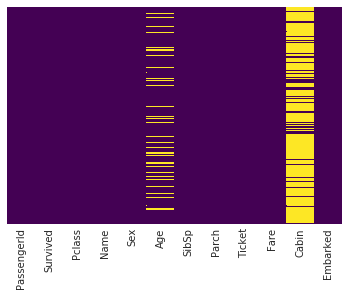Roughly 20 percent of the Age data is missing. The proportion of Age missing is likely small enough for reasonable replacement with some form of imputation. Looking at the Cabin column, it looks like we are just missing too much of that data to do something useful with at a basic level.

In :
#people who survived v/s who didn't

sns.set_style('whitegrid')
sns.countplot(x='Survived', data= titanic, palette='RdBu_r')

Out:
<matplotlib.axes._subplots.AxesSubplot at 0x7fbc3d4d0358>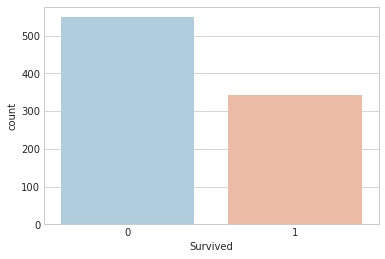In :
sns.countplot(x='Survived', hue='Sex', data= titanic, palette='RdBu_r')

Out:
<matplotlib.axes._subplots.AxesSubplot at 0x7fbc3d422ef0>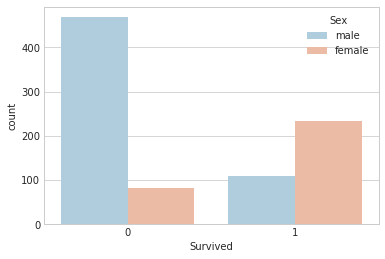In :
sns.countplot(x='Survived', hue='Pclass', data= titanic, palette='rainbow')

Out:
<matplotlib.axes._subplots.AxesSubplot at 0x7fbc3d356c18>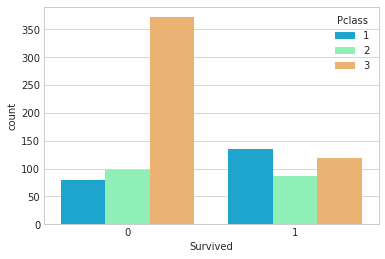In :
#looking at the description of the dataset
titanic.describe()

Out:
PassengerId Survived Pclass Age SibSp Parch Fare
count 891.000000 891.000000 891.000000 714.000000 891.000000 891.000000 891.000000
mean 446.000000 0.383838 2.308642 29.699118 0.523008 0.381594 32.204208
std 257.353842 0.486592 0.836071 14.526497 1.102743 0.806057 49.693429
min 1.000000 0.000000 1.000000 0.420000 0.000000 0.000000 0.000000
25% 223.500000 0.000000 2.000000 20.125000 0.000000 0.000000 7.910400
50% 446.000000 0.000000 3.000000 28.000000 0.000000 0.000000 14.454200
75% 668.500000 1.000000 3.000000 38.000000 1.000000 0.000000 31.000000
max 891.000000 1.000000 3.000000 80.000000 8.000000 6.000000 512.329200
In :
sns.distplot(titanic['Age'].dropna(),color='darkred',bins=30)

Out:
<matplotlib.axes._subplots.AxesSubplot at 0x7fbc3d3a7320>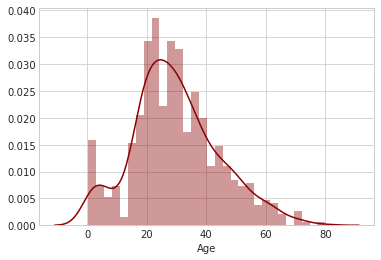The distribution plot for age is slightly right skewed. There is not much problem of outliers as such.

In :
titanic['Fare'].hist(color='green',bins=40,figsize=(8,4))

Out:
<matplotlib.axes._subplots.AxesSubplot at 0x7fbc3d169630>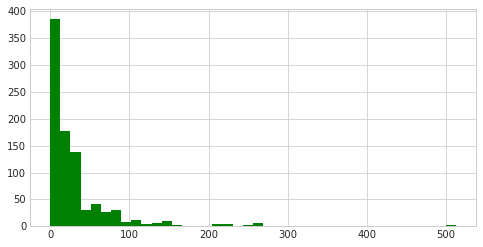We have a few outliers in the fare section. We can ignore them while training our model

## Data Cleaning¶

Lets fill in the missing values of the age column. We can do this by taking mean of the age. Smarter way will be to fill in the missing blanks with the mean of the Pclass they belong too.

In :
plt.figure(figsize=(12, 7))
sns.boxplot(x='Pclass',y='Age',data=titanic,palette='winter')

Out:
<matplotlib.axes._subplots.AxesSubplot at 0x7fbc3d0302e8>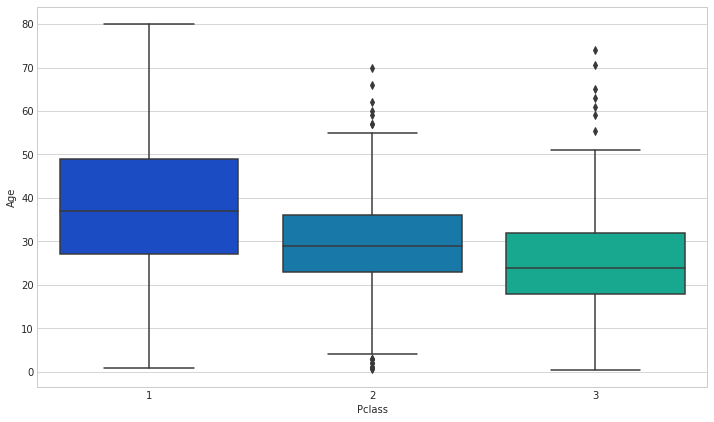We can see the wealthier passengers in the higher classes tend to be older, which makes sense. We'll use these average age values to impute based on Pclass for Age.

In :
def impute_age(cols):
Age = cols
Pclass = cols

if pd.isnull(Age):

if Pclass == 1:
return 37
elif Pclass == 2:
return 29
else:
return 24
else:
return Age

In :
titanic['Age'] = titanic[['Age', 'Pclass']].apply(impute_age, axis = 1)


Let's check the heatmap again

In :
sns.heatmap(titanic.isnull(), yticklabels=False, cbar=False, cmap='viridis')

Out:
<matplotlib.axes._subplots.AxesSubplot at 0x7fbc3d08fcc0>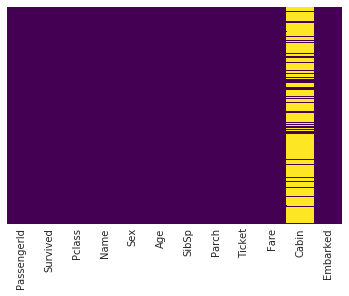Let's go ahead and give 1 tag to value with valid Cabin no. and 0 tag to value with NaN.

In :
def impute_cabin(col):

Cabin = col

if type(Cabin) == str:
return 1
else:
return 0

In :
titanic['Cabin'] = titanic[['Cabin']].apply(impute_cabin, axis = 1)


Let's check the heatmap again

In :
sns.heatmap(titanic.isnull(), yticklabels=False, cbar=False, cmap='viridis')

Out:
<matplotlib.axes._subplots.AxesSubplot at 0x7fbc3cf4aeb8>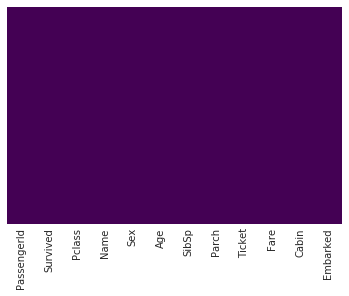In :
titanic.head()

Out:
PassengerId Survived Pclass Name Sex Age SibSp Parch Ticket Fare Cabin Embarked
0 1 0 3 Braund, Mr. Owen Harris male 22.0 1 0 A/5 21171 7.2500 0 S
1 2 1 1 Cumings, Mrs. John Bradley (Florence Briggs Th... female 38.0 1 0 PC 17599 71.2833 1 C
2 3 1 3 Heikkinen, Miss. Laina female 26.0 0 0 STON/O2. 3101282 7.9250 0 S
3 4 1 1 Futrelle, Mrs. Jacques Heath (Lily May Peel) female 35.0 1 0 113803 53.1000 1 S
4 5 0 3 Allen, Mr. William Henry male 35.0 0 0 373450 8.0500 0 S
In :
titanic.dropna(inplace=True)


We'll need to convert categorical features to dummy variables using pandas! Otherwise our machine learning algorithm won't be able to directly take in those features as inputs.

In :
titanic.info()

<class 'pandas.core.frame.DataFrame'>
Int64Index: 889 entries, 0 to 890
Data columns (total 12 columns):
PassengerId    889 non-null int64
Survived       889 non-null int64
Pclass         889 non-null int64
Name           889 non-null object
Sex            889 non-null object
Age            889 non-null float64
SibSp          889 non-null int64
Parch          889 non-null int64
Ticket         889 non-null object
Fare           889 non-null float64
Cabin          889 non-null int64
Embarked       889 non-null object
dtypes: float64(2), int64(6), object(4)
memory usage: 90.3+ KB

In :
#Let's work on a copy of our present dataset for further operations

dataset = titanic

In :
sex = pd.get_dummies(dataset['Sex'],drop_first=True)
embark = pd.get_dummies(dataset['Embarked'],drop_first=True)

dataset.drop(['Sex','Embarked','Name','Ticket'],axis=1,inplace=True)

dataset = pd.concat([dataset,sex,embark],axis=1)

In :
dataset.head()

Out:
PassengerId Survived Pclass Age SibSp Parch Fare Cabin male Q S
0 1 0 3 22.0 1 0 7.2500 0 1 0 1
1 2 1 1 38.0 1 0 71.2833 1 0 0 0
2 3 1 3 26.0 0 0 7.9250 0 0 0 1
3 4 1 1 35.0 1 0 53.1000 1 0 0 1
4 5 0 3 35.0 0 0 8.0500 0 1 0 1

## Building regression models¶

Let's start by splitting our data into a training set and test set

In :
#Train Test Split

from sklearn.model_selection import train_test_split

In :
X_train, X_test, y_train, y_test = train_test_split(dataset.drop('Survived',axis=1),
dataset['Survived'], test_size=0.25,
random_state=101)


## Training and Predicting¶

In :
from sklearn.linear_model import LogisticRegression


Using Logistic Regression

In :
regressor = LogisticRegression()
regressor.fit(X_train, y_train)

Out:
LogisticRegression(C=1.0, class_weight=None, dual=False, fit_intercept=True,
intercept_scaling=1, max_iter=100, multi_class='ovr', n_jobs=1,
penalty='l2', random_state=None, solver='liblinear', tol=0.0001,
verbose=0, warm_start=False)
In :
pred = regressor.predict(X_test)


### Let's evaluate¶

In :
from sklearn.metrics import classification_report, confusion_matrix, accuracy_score, log_loss

In :
print(classification_report(y_test, pred))
print('\n')
print(confusion_matrix(y_test, pred))
print('\n')
print(accuracy_score(y_test, pred))

             precision    recall  f1-score   support

0       0.81      0.91      0.86       136
1       0.83      0.67      0.74        87

avg / total       0.82      0.82      0.81       223

[[124  12]
[ 29  58]]

0.816143497758


Using SVM

In :
from sklearn.svm import SVC

In :
regressor2 = SVC()
regressor2.fit(X_train, y_train)

Out:
SVC(C=1.0, cache_size=200, class_weight=None, coef0=0.0,
decision_function_shape='ovr', degree=3, gamma='auto', kernel='rbf',
max_iter=-1, probability=False, random_state=None, shrinking=True,
tol=0.001, verbose=False)
In :
pred2 = regressor2.predict(X_test)

In :
print(classification_report(y_test, pred2))
print('\n')
print(confusion_matrix(y_test, pred2))
print('\n')
print(accuracy_score(y_test, pred2))

             precision    recall  f1-score   support

0       0.61      0.99      0.75       136
1       0.00      0.00      0.00        87

avg / total       0.37      0.60      0.46       223

[[134   2]
[ 87   0]]

0.600896860987


Using K-NN

In :
from sklearn.neighbors import KNeighborsClassifier

In :
regressor3 = KNeighborsClassifier(n_neighbors=5)
regressor3.fit(X_train, y_train)

Out:
KNeighborsClassifier(algorithm='auto', leaf_size=30, metric='minkowski',
metric_params=None, n_jobs=1, n_neighbors=5, p=2,
weights='uniform')
In :
pred3 = regressor3.predict(X_test)

In :
print(classification_report(y_test, pred3))
print('\n')
print(confusion_matrix(y_test, pred3))
print('\n')
print(accuracy_score(y_test, pred3))

             precision    recall  f1-score   support

0       0.67      0.78      0.72       136
1       0.53      0.39      0.45        87

avg / total       0.61      0.63      0.61       223

[[106  30]
[ 53  34]]

0.627802690583


In :
from sklearn.ensemble import AdaBoostClassifier

In :
regressor4 = AdaBoostClassifier()
regressor4.fit(X_train, y_train)

Out:
AdaBoostClassifier(algorithm='SAMME.R', base_estimator=None,
learning_rate=1.0, n_estimators=50, random_state=None)
In :
pred4 = regressor4.predict(X_test)

In :
print(classification_report(y_test, pred4))
print('\n')
print(confusion_matrix(y_test, pred4))
print('\n')
print(accuracy_score(y_test, pred4))

             precision    recall  f1-score   support

0       0.83      0.85      0.84       136
1       0.76      0.74      0.75        87

avg / total       0.81      0.81      0.81       223

[[116  20]
[ 23  64]]

0.807174887892